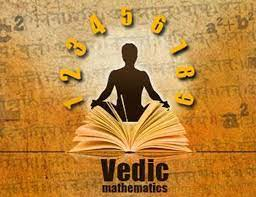Choose a Course Pricing Plan

Vedic Maths

Vedic Mathematics was rediscovered from the Hindu sect oldest scriptures Vedas,
Vedic mathematics works on certain principles that develops our mental reasoning ability by simplifying the mathematical calculations easier to understand.

If you are someone who loves numbers, this is the perfect course to make you fall in love with maths!

Who will be beneficial

who find it difficult to quickly perform mathematical computations, or who wish to find easier and less time-consuming techniques to perform mathematical calculations..

What you'll learn

An introduction to basic techniques and methods used in Vedic Mathematics to help improve your aptitude for Maths and learn one line mental calculations.

Description

Without mathematics, there’s nothing one can do. Everything around us is mathematics or derived from. Mathematics. The entire system of computer or super computer are numbers 0 and 1.

Who this course is for

All who find it difficult to quickly perform mathematical computations, or who wish to find easier and less time-consuming techniques to perform big and complex mathematical calculations.

Curriculam

Introduction

Math for Data

Why study Vedic Mathematics

Complements of
numbers

Multiplication of Complementary numbers

Multiplication of a number by pattern of 9s

Special pattern multiplication for two digit numbers

Base multiplication below base 10

Base multiplication above base 10

Base multiplication below base 100

Base multiplication above base 100

Base multiplication below base 1000

Base multiplication above base 1000

Multiplication of a number with 5

Multiplication of a number with 25

Multiplication of a number with 125

Multiplication with 11

Multiplication with 12

Multiplication with 15

Squaring a number ending in 5

Squaring a number above the base

Squaring a number below the base

Squaring any two digit number

Multiplication of any two digit number

Your E-mail Address Will not be published. Required field are marked *

Name*
E-mail*

No Video Found

### Information

• Seats: 10
• Age: 6+
• Duration: 1 year
• Course Type: online
• Video Available: No